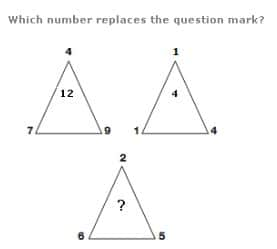Riddle: Which Number Replaces The Question Mark In The 3rd Triangle - Examined Existence

# Riddle: Which Number Replaces The Question Mark In The 3rd TriangleHere’s the riddle. There’s 3 triangles below. All numbers are given except for the question mark. What number replaces the question mark?• Riddle

Which number replaces the question mark of the third triangle.  Use clues from the first two triangles to figure out the answer.

By Tri
By Tri
By Tri
By Tri